# A ball is thrown vertically up (taken as + z-axis) from the ground.

Question:

A ball is thrown vertically up (taken as $+$ z-axis) from the ground. The correct momentum-height ( $\mathrm{p}-\mathrm{h}$ ) diagram is:

1.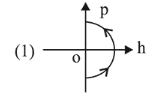2.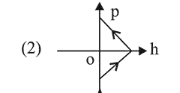3.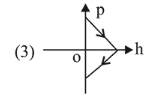4.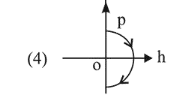Correct Option: , 4

Solution:

(4) $v^{2}=u^{2}-2 g h$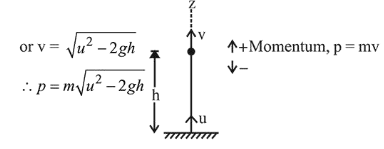Therefore graph between $\mathrm{p}$ and $\mathrm{h}$ cannot have straight line.

(2) and (3) are not possible.

During upward journey as $\mathrm{h}$ increases, $\mathrm{p}$ decreases and in downward journey as $\mathrm{h}$ decreases $\mathrm{p}$ increases. Therefore 4 is the correct option.